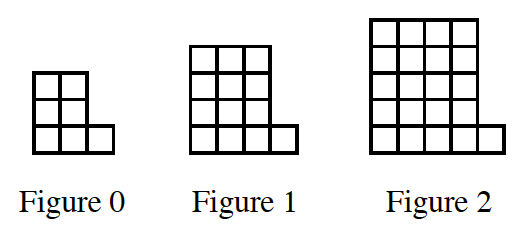### Home > CCA > Chapter Ch11 > Lesson 11.2.1 > Problem11-36

11-36.

Find an equation that represents the number of tiles in the tile pattern below. Homework Help ✎The shapes are rectangles with one extra tile.
What is the formula for the area of a rectangle?

One possible equation is
$(x+2)(x+3)+1=y$, or
$x^2+5x+7=y$.